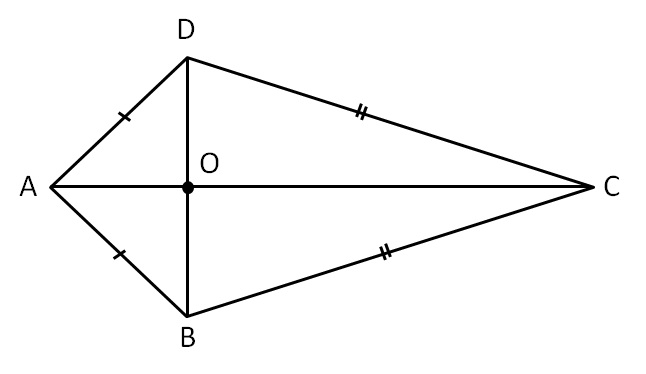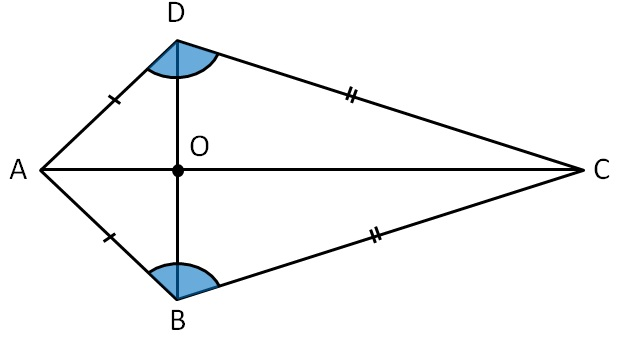Chapter 3 Class 8 Understanding Quadrilaterals
Concept wiseTwo pairs of consecutive sides are equal

i.e. AB = AD & BC = DC

Properties of Kite:

1. Diagonals are perpendicular to each other
AC ⊥ BD2. One diagonal bisects the other
i.e. AC bisects BD
⇒ BO = DO

1. One pair of opposite angle is equal
∠ B = ∠ D
but ∠ A ≠ ∠ C

Get live Maths 1-on-1 Classs - Class 6 to 12